# Measurement Conversions Ratios Unit Analysis Lesson Plan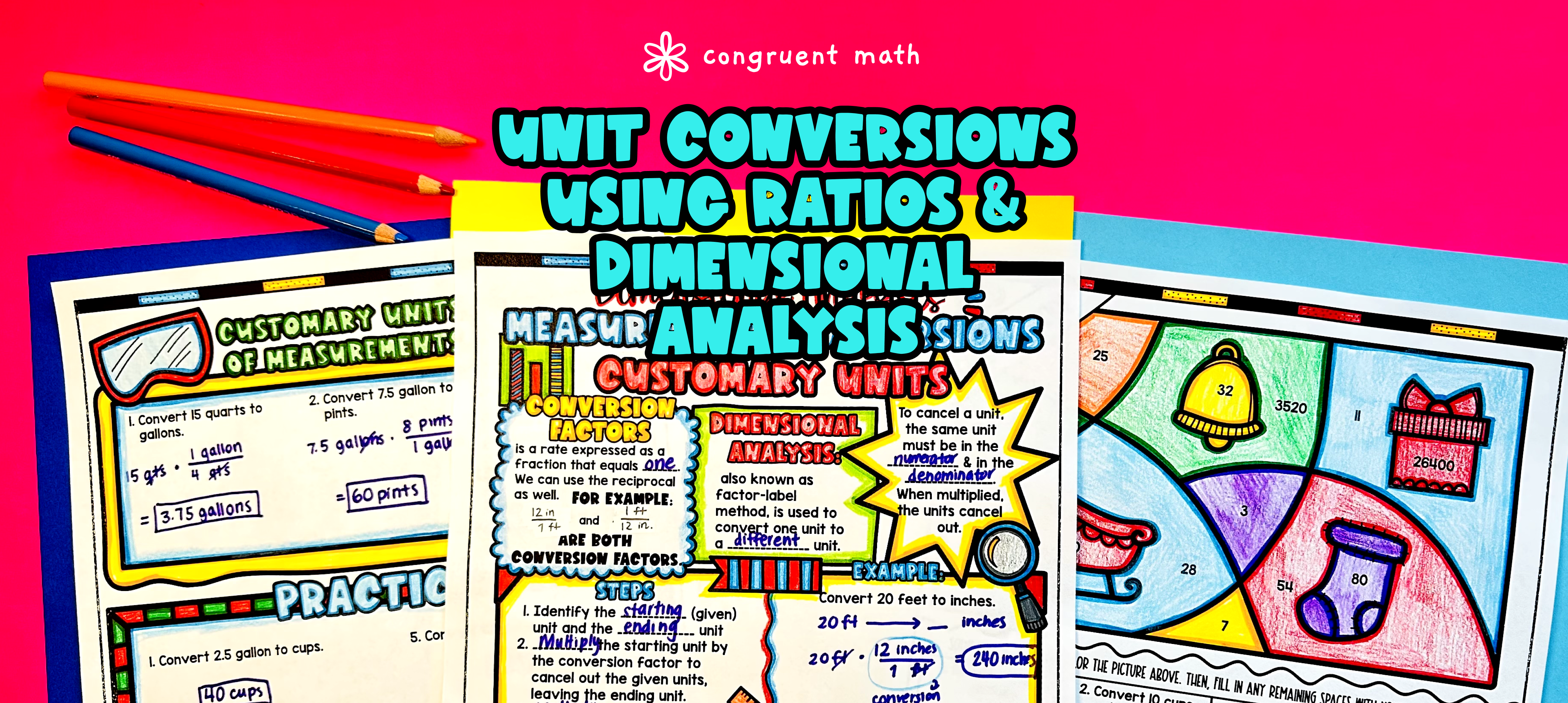Pin This

## Overview

Ever wondered how to teach measurement conversions in an engaging way to your 6th grade students?

In this lesson plan, students will learn about measurement conversions using ratios and dimensional analysis. Through artistic and interactive guided notes, check for understanding activities, a practice color by number worksheet, and a maze worksheet, students will gain a comprehensive understanding of measurement conversions.

The lesson culminates with a real-life application where students will read and write about real-life uses of unit conversions. Students will explore how measurement conversions are used in everyday situations, making the topic more relatable and applicable to their lives.

## Get the Lesson Materials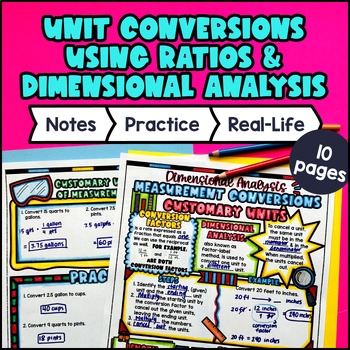\$4.25

## Learning Objectives

After this lesson, students will be able to:

• Convert measurements between different units using ratios and dimensional analysis

• Use conversion factors and ratios to accurately convert measurements in customary units

• Explain real life applications of unit measurement conversions

## Prerequisites

Before this lesson, students should be familiar with:

• Knowledge of the basic operations of addition, subtraction, multiplication, and division of whole numbers and decimal numbers

• Understanding of the concept of equivalent fractions and how to simplify fractions (optional, but helpful)

## Key Vocabulary

• Measurement Conversions

• Ratios

• Dimensional Analysis

• Customary Units

## Procedure

### Introduction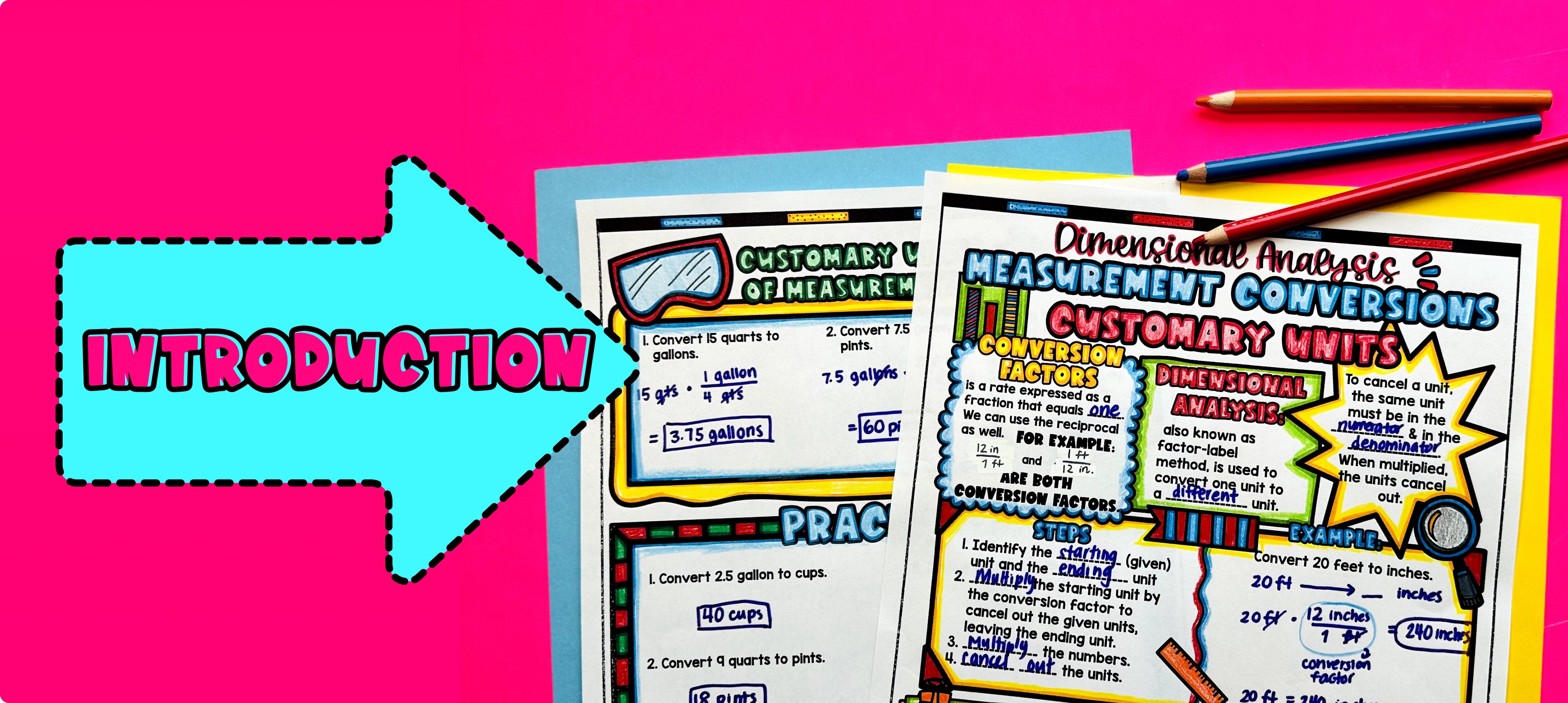Pin This

As a hook, ask students why measurement conversions are important in real-life situations. Refer to the last page of the guided notes as well as the FAQs below for ideas.

Use the first page of guided notes to introduce the concept of measurement conversions using ratios and dimensional analysis. Walk through the key points of how to convert between different units using ratios and conversion factors.

Based on student responses, reteach any concepts that students need extra help with. If your class has a wide range of proficiency levels, you can pull out students for reteaching, and have more advanced students begin working on the practice exercises.

### Practice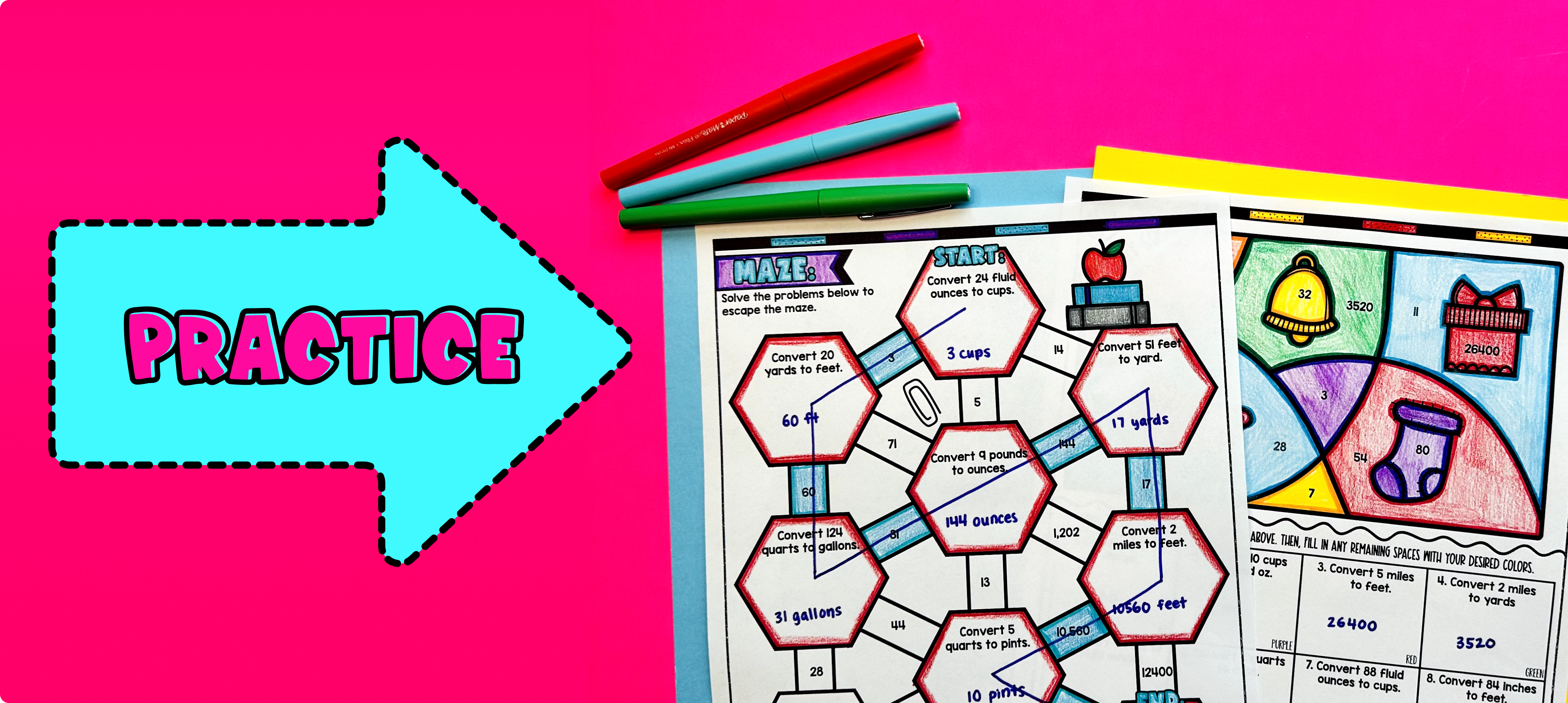Pin This

Have students practice measurement conversions using ratios and dimensional analysis using the practice worksheet included in the resource (page 2 of guided notes). Walk around the classroom to answer any questions students may have.

Fast finishers can then work on the additional practice activities provided in the resource, such as the color by code or maze (page 3 and page 4 of guided notes). You can assign these activities as homework for the remainder of the class.

### Real-Life Application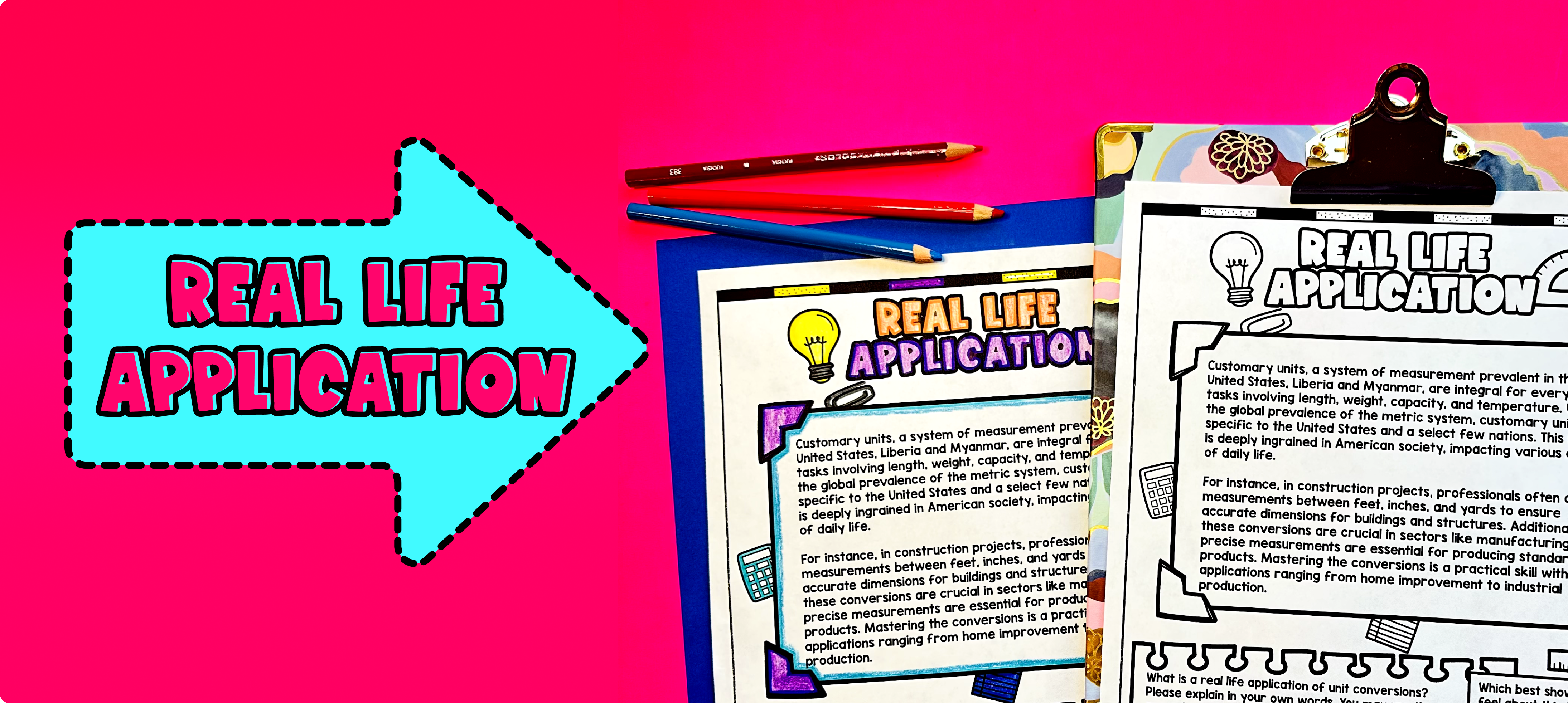Pin This

Use the last page of the guided notes to bring the class back together, and introduce the concept of real-life applications of unit conversions. Explain to students that unit conversions are not just useful in math class, but they are also used in many real-world scenarios.

Give examples of real-life situations where unit conversions are necessary, such as:

• Converting miles to kilometers when traveling internationally.

• Converting temperature from Fahrenheit to Celsius when cooking or baking.

• Converting currency when traveling to a different country.

• Converting units of measurement when shopping, such as converting ounces to pounds or gallons to liters.

Encourage students to think about other situations where they may need to use unit conversions in their daily lives.

Refer to the FAQ section of the teaching resource for more ideas on how to incorporate real-life applications of unit conversions into your lesson.